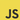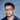# How to build a 3D Renderer with 2D HTML Canvas

These last few months I've taken a deeper interest in math, physics, and art. I wanted to make a fun and interesting project. So I took inspiration from something I learned in art class along time ago - Vanishing Points. I built a 3D cube representation in HTML canvas using plain javascript. This tutorial below will show you how to create one as well.

#### Prerequisites

• Basic knowledge of HTML,CSS, and Javascript
• High School Geometry
• Vanishing Points

#### Goals

The goal is to learn how to create a 3D representation of a cube on a 2D HTML canvas, using a single vanishing point. This guide is a multistep blog post series, the first demo will show a much simpler example## Planning

Breaking things into small tasks will make things easier to understand.

• Initialize Canvas
• Initialize points in space
• Draw two lines pointing away from the cube
• Add event listeners for toggling variables

There's many other ways of building this cube, but this is how we're going to do it here. Building a vanishing point cube could be done in many other ways with pure CSS, SVGs, or even a webGL library like three.js

## Setup

First create the basic setup. This is the `index.html` file starting point with a canvas element to modify

``````<!DOCTYPE html>
<html>

<body>
<canvas></canvas>
<script src="script.js"></script>
</body>

</html>``````

In the same folder add some basic styles in `style.css`. This positions any sliders or overlays to be above the canvas when written in HTML

``````body {
background-color: lightgray;
display: flex;
justify-content: center;
align-items: center;
}
.slideContainer {
position: fixed;
right: 30px;
top: 30px;
background-color: lightblue;
z-index: 20;
}
canvas {
border: 1px dotted red;
background-color: lightgray;
transform: scale(0.5);
}``````

### Initialize Canvas

The first thing we want to do is create a single point in space. To do this, you need to select the `canvas` object using a query selector. Since `canvas` elements work by pixel values, we'll change values on those units. We're going to use a coordinate grid system of 1600 wide x 800 high.

Note that X grows in size going right, y gets larger growing downward. (0,0) starts off at the topleft of screen

``````var canvas = document.querySelector("canvas");

canvas.width = window.innerWidth;
canvas.height = window.innerHeight;

canvas.width = 1600;
canvas.height = 800;

var ctx = canvas.getContext("2d");``````

### Initialize points in space

We'll drawn an origin point. For this example, we need 3 points. The origin point, and two points in space to build a vanishing point line. We'll also need to define an initial size for the cube. Cubes are all the same length on every side, I defined it with a variable called `delta`

``````// Vanishing point 0
var x0 = 400;
var y0 = 400;

// Vanishing point end 0a
var x0a = 0;
var y0a = 800;

// Vanishing point end 0b
var x0b = 800;
var y0b = 800;

// Length of box's side
var delta = 700;``````

### Draw two lines pointing away from the cube

Since we have 3 points in space, we can start drawing lines. We use the canvas objects method called `beginPath()` to tell it to draw a line. After that, we specify a starting point, an endpoint, and a stroke style / value.

``````// First Line
ctx.beginPath();
ctx.moveTo(x0, y0);
ctx.lineTo(x0a, y0a);
ctx.strokeStyle = 'red';
ctx.stroke();

// Second Line
ctx.beginPath();
ctx.moveTo(x0, y0);
ctx.lineTo(x0b, x0b);
ctx.strokeStyle = 'green';
ctx.stroke();``````

At this point, you should have a simple canvas element with 2 lines on it. Check out the javascript file code below to see where we are at.

### Draw a Front Face

The frontface of a cube is a square, where all sides are equal. We'll use the end of the right line as one of the four points of the square.

``````// Box based on second Line
ctx.beginPath();
ctx.moveTo(x0b, y0b); // starting point
ctx.lineTo(x0b + delta, y0b); // right x+100
ctx.lineTo(x0b + delta, y0b - delta); // up y-100
ctx.lineTo(x0b, y0b - delta); // left x-100
ctx.lineTo(x0b, y0b); // down y+100``````

### Draw lines from Front Face Vertices to Origin

What we're doing here is just figuring out new points for the square, using `delta` as the length of one side of the square. We can add these few lines to connect two of the vertices to the origin point:

``````ctx.lineTo(x0b, y0b - delta); // back up y-100
ctx.lineTo(x0, y0);
ctx.lineTo(x0b + delta, y0b - delta);
ctx.strokeStyle = 'blue';
ctx.stroke();``````

Before we finish up here, take all the `ctx._____` things you wrote and wrap it inside of a function. This is necessary because we are going to call the function everytime we modify an input value. The logic should look like so:

``````function init(){
// ctx.____ logic we just wrote
}

init();``````

We want to have a canvas element that dynamically rerenders on input. Add a `slideContainer` with the `input` inside of it next to `canvas` element.

``````<div class="slideContainer">
<input type="range" min="1" max="800" value="50" class="slider" id="myRange">
</div>``````

We'll also have an event listener targeting the slider we created. It will do the following: clear any previous drawn lines, update a global variable `delta`, and redraw the entire canvas. Remember `function init()`? This is where we call it once we use our slider

``````slider.oninput = function () {
ctx.clearRect(0, 0, canvas.width, canvas.height);
delta = +this.value;
requestAnimationFrame(init());
}``````

Check the following code to see the entire `script.js` file up to this point. See the codepen demo to see everything we did thus far.

### Summary

In the next part I will cover how to refactor this, and create the original gif from the start. We'll add additional slider controls# Hi 👋I'm Vincent Tang, a web developer specializing in modern Javascript. This website is my digital garden of notes on backend, frontend, and devops! I'm the founder of Tampa Devs and I also run a coding podcast called Code Chefs